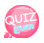# Validate Email

Let's learn how to validate your email.
Use the filter_Var() built-in function.
And use the constant FILTER_VALIDATE_EMAIL as an argument.

## How to validate email using filter_Var() function

```filter_Var('email address',FILTER_VALIDATE_EMAIL);
```

The first argument uses an email address and the second uses the constant FILTER_VALIDATE_EMAIL.
As shown in the example, you can change the purpose according to the value of a constant.
Returns true if the email address is valid or false.
The following example validates an email.

```<?php
\$email = "mybookforweb@gmail.com";

\$checkMail = filter_Var(\$email, FILTER_VALIDATE_EMAIL);

if(\$checkMail == true) {
echo "correct email";
} else {
}
?>```

the result of this code```<?php
\$email = "mybookforweb@";

\$checkMail = filter_Var(\$email, FILTER_VALIDATE_EMAIL);

if(\$checkMail == true) {
echo "correct email";
} else {